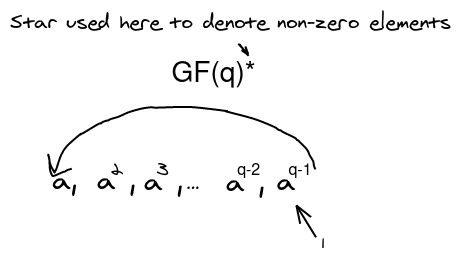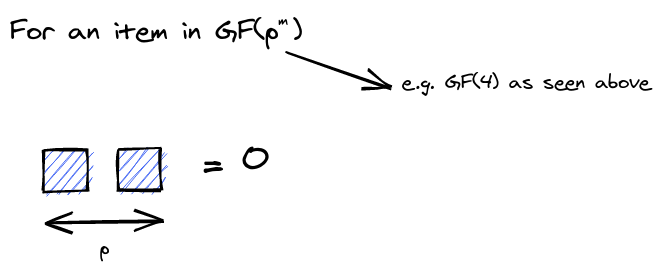# Finite Fields

## CaC

A group must follow the following rules

Associativity: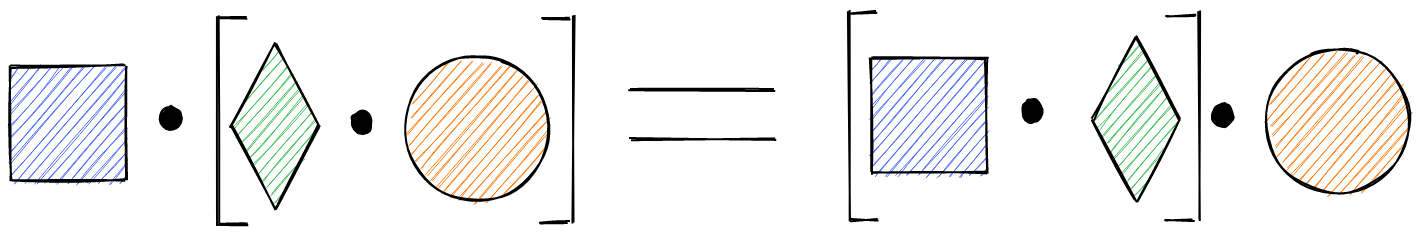Identity element: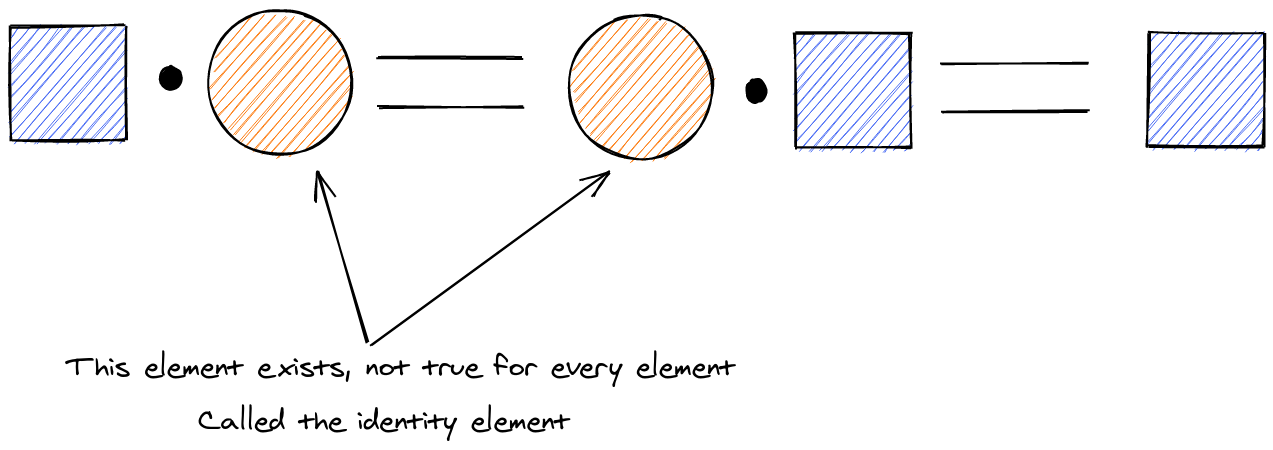Inverses: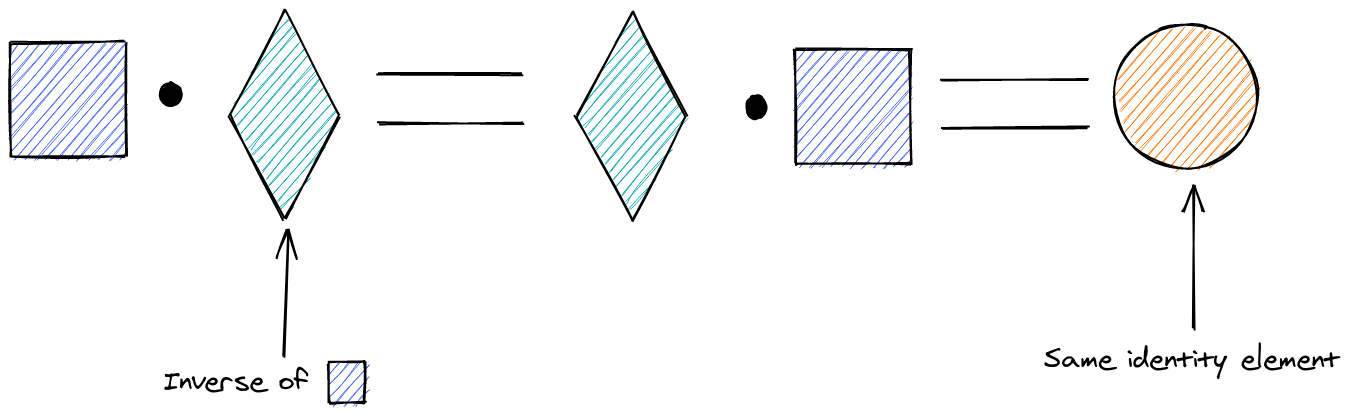## Abelian group

An abelian group is also commutative: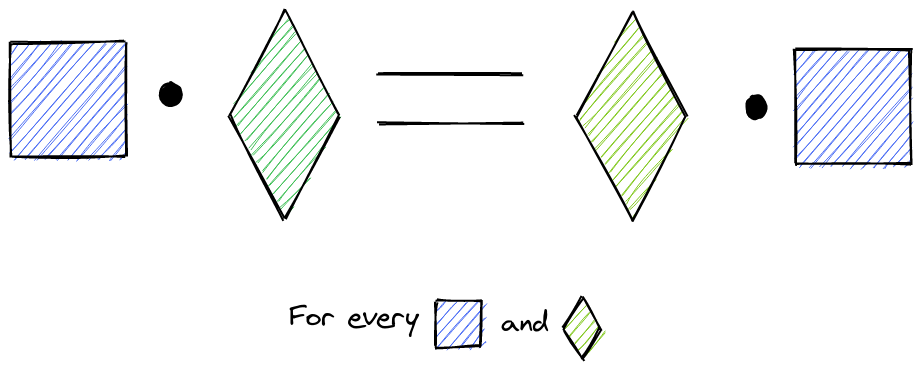## Field

A field is a 5-tuple

$$(F, +, \times, 0, 1)$$

with

1. $(F, +)$ being an Abelian group with identity 0

e.g. $1+0=0+1=1$

2. $(F*, \times)$ being an Abelian group with identity 1

e.g. $2\times 1=1\times 2 = 2$

3. Multiplication distributes over the addition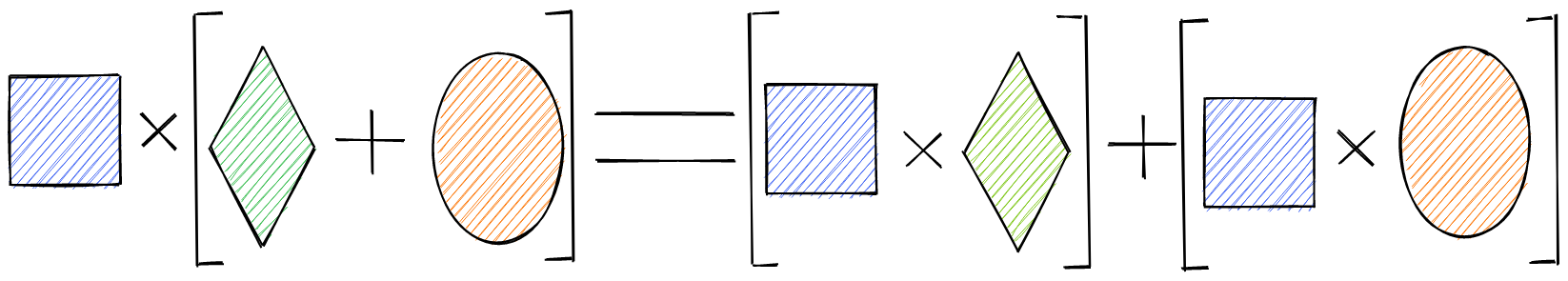## Galois field

Theorem

Any finite field is of order $q=p^m$, where p is a prime number and m is an integer $\geqslant 1$. All fields of a given size are the same.

These have two different notations that mean the same thing, $GF(q)$ and $\mathbb{F}_q$

## Extension field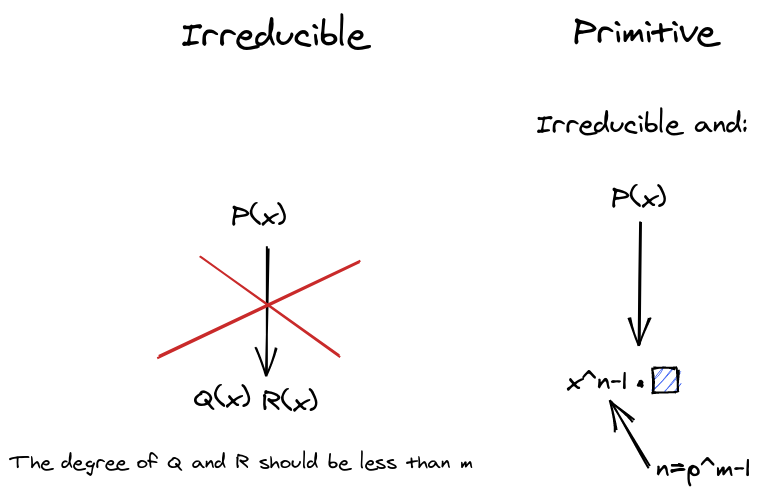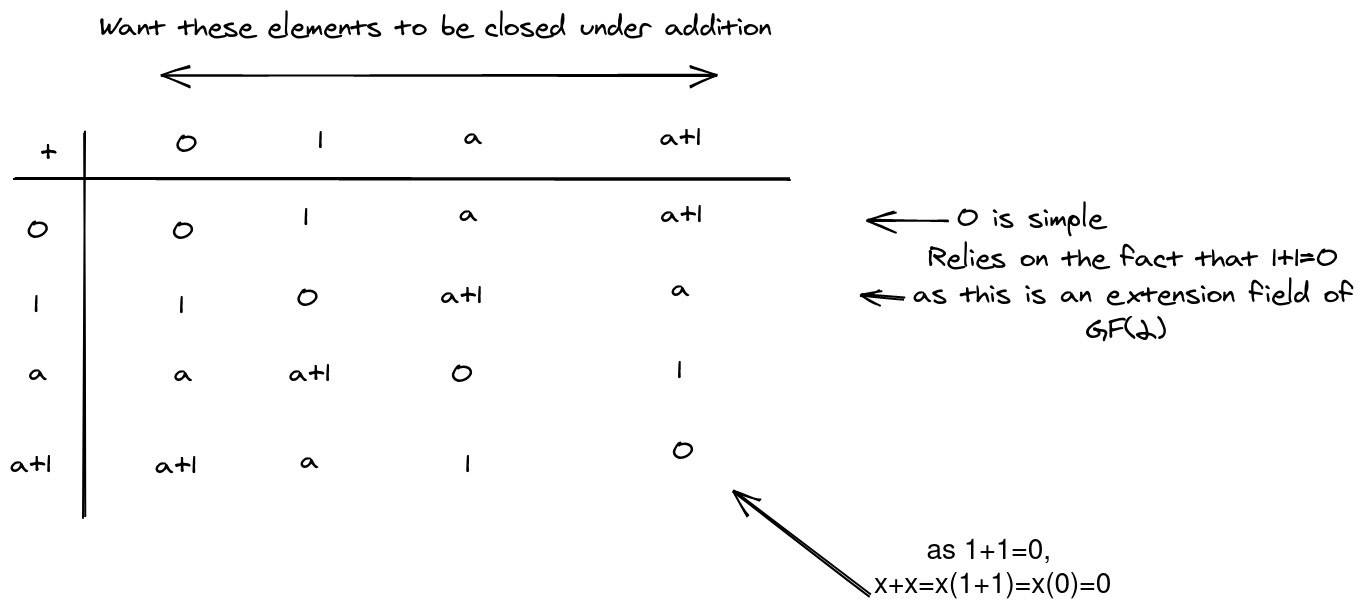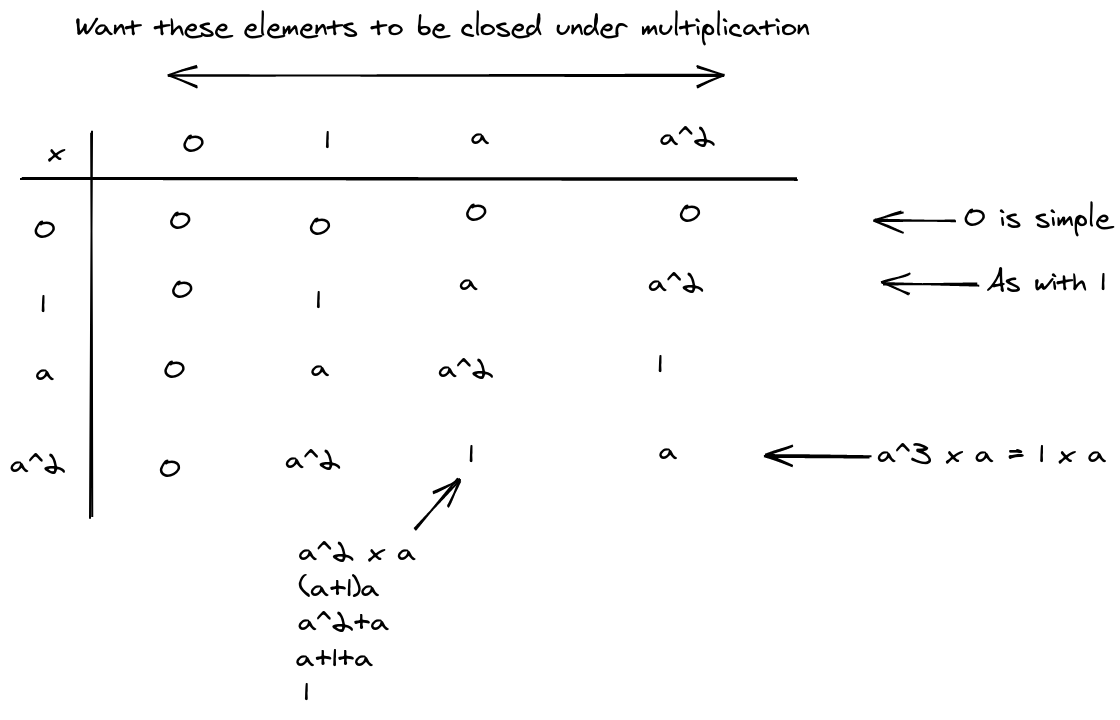### Properties

Multiplicative structure